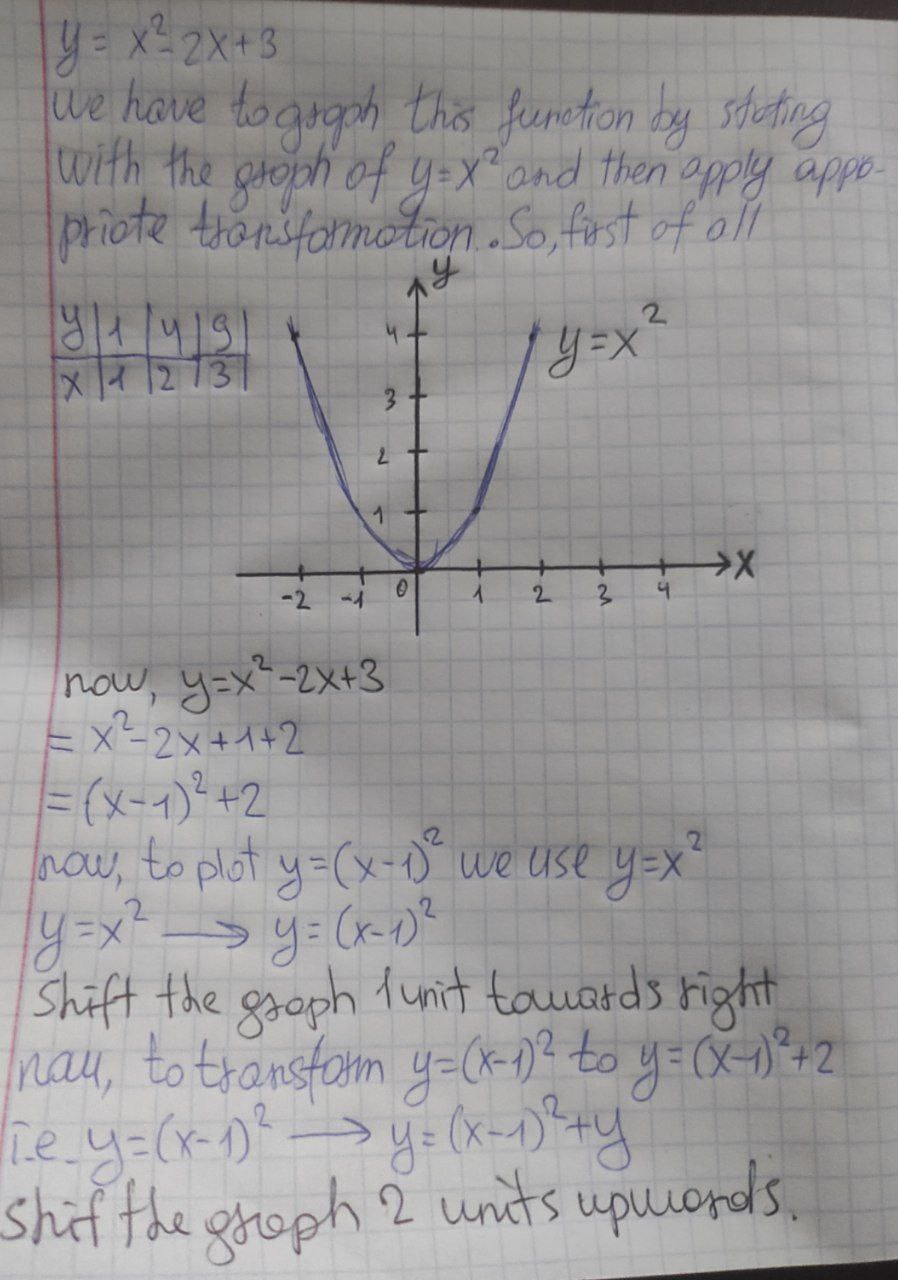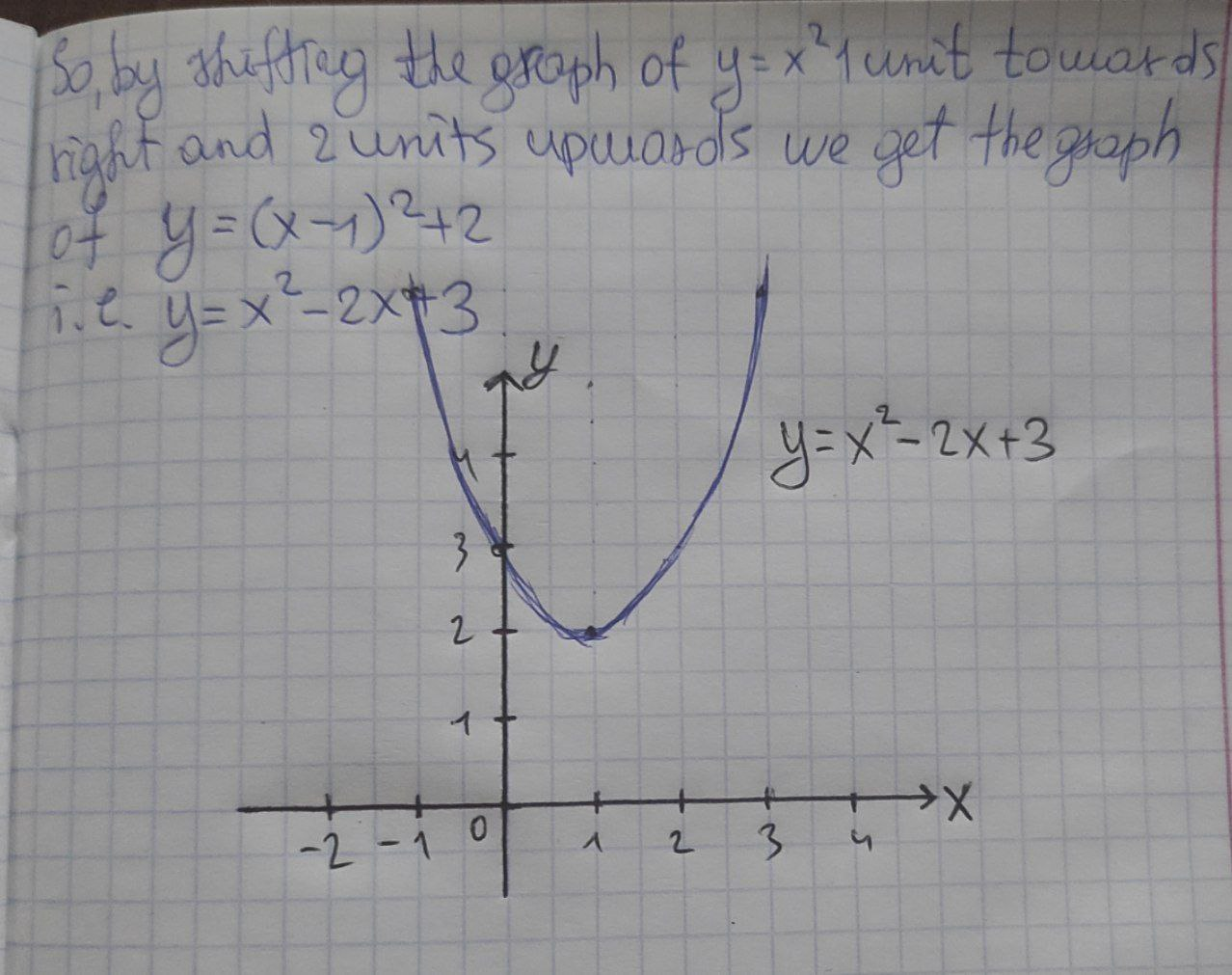# )Graph the function y = x^2&minus; 2x +

)Graph the function y = x^2− 2x + 3, not by plotting points, but by starting with the graph of y = x^2 and then applying the appropriate transformations. Describe your transformations
You can still ask an expert for help

• Questions are typically answered in as fast as 30 minutes

Solve your problem for the price of one coffee

• Math expert for every subject
• Pay only if we can solve itquestion2answer